# C++ Pipes

C++ Pipes

By Jonathan Boccara

Expressive code can make life easier. Jonathan Boccara demonstrates fluent pipelines for collections in C++.

Pipes are small components for writing expressive code when working on collections. Pipes chain together into a pipeline that receives data from a source, operates on that data, and sends the results to a destination.

This is a header-only library, implemented in C++14.

The library is under development and subject to change. Contributions are welcome. You can also log an issue if you have a wish for enhancement or if you spot a bug.

## A first example

Here is a simple example of a pipeline made of two pipes: ``` transform ``` and ``` filter ``` :

```  auto const source = std::vector<int>{0, 1, 2, 3,
4, 5, 6, 7, 8, 9};
auto destination = std::vector<int>{};
source >>= pipes::filter([](int i)
{ return i % 2 == 0; })
>>= pipes::transform([](int i)
{ return i * 2; })
>>= pipes::push_back(destination);
// destination contains {0, 4, 8, 12, 16};```

### What’s going on here

• Each element of ``` source ``` is sent to ``` filter ``` .
• Every time ``` filter ``` receives a piece of data, it sends its to the next pipe (here, ``` transform ``` ) only if that piece of data satisfies ``` filter ``` ’s predicate.
• ``` transform ``` then applies its function on the data its gets and sends the result to the next pipe (here, ``` pipes::push_back ``` ).
• ``` pipes::push_back ``` ``` push_back ``` s the data it receives to its ``` vector ``` (here, ``` destination ``` ).

## A second example

Here is a more elaborate example with a pipeline that branches out in several directions:

```  A >>= pipes::transform(f)
>>= pipes::filter(p)
>>= pipes::unzip(pipes::push_back(B),
pipes::demux(pipes::push_back(C),
pipes::filter(q) >>= pipes::push_back(D),
pipes::filter(r) >>= pipes::push_back(E));```

Here, ``` unzip ``` takes the ``` std::pair ``` s or ``` std::tuple ``` s it receives and breaks them down into individual elements. It sends each element to the pipes it takes (here ``` pipes::push_back ``` and ``` demux ``` ).

``` demux ``` takes any number of pipes and sends the data it receives to each of them.

Since data circulates through pipes, real life pipes and plumbing provide a nice analogy (which gave its names to the library). For example, the above pipeline can be graphically represented like Figure 1.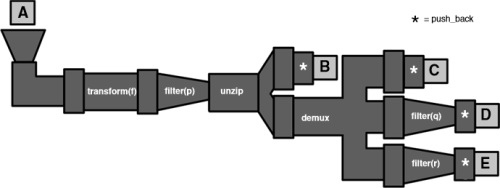Figure 1

## Doesn’t it look like ranges?

Pipes sort of look like ranges adaptors from afar, but those two libraries have very different designs.

Range views are about adapting ranges with view layers, and reading through those layers in lazy mode. Pipes are about sending pieces of data as they come along in a collection through a pipeline, and letting them land in a destination.

Ranges and pipes have overlapping components such as ``` transform ``` and ``` filter ``` . But pipes do things like ranges can’t do, such as ``` pipes::mux ``` , ``` pipes::demux ``` and ``` pipes:unzip ``` , and ranges do things that pipes can’t do, like infinite ranges.

It is possible to use ranges and pipes in the same expression though:

```  ranges::view::zip(dadChromosome, momChromosome)
>>= pipes::transform(crossover) // crossover

// takes and returns a tuple of 2 elements
>>= pipes::unzip(pipes::push_back
(gameteChromosome1),
pipes::push_back(gameteChromosome2));```

## Operating on several collections

The pipes library allows to manipulate several collections at the same time, with the ``` pipes::mux ``` helper. Note that contrary to ``` range::view::zip ``` , ``` pipes::mux ``` doesn’t require to use tuples.

```  auto const input1 = std::vector<int>{1, 2, 3, 4,
5};
auto const input2 = std::vector<int>{10, 20, 30,
40, 50};
auto results = std::vector<int>{};

pipes::mux(input1, input2)
>>= pipes::filter   ([](int a, int b)
{ return a + b < 40; })
>>= pipes::transform([](int a, int b)
{ return a * b; })
>>= pipes::push_back(results);```

// results contains {10, 40, 90}

### Operating on all the possible combinations between several collections

``` pipes::cartesian_product ``` takes any number of collections, and generates all the possible combinations between the elements of those collections. It sends each combination successively to the next pipe after it.

Like ``` pipes::mux ``` , ``` pipes::cartesian_product ``` doesn’t use tuples but sends the values directly to the next pipe:

```  auto const inputs1 = std::vector<int>{1, 2, 3};
auto const inputs2
= std::vector<std::string>{"up", "down"};
auto results = std::vector<std::string>{};

pipes::cartesian_product(inputs1, inputs2)
>>= pipes::transform([](int i,
std::string const& s)
{ return std::to_string(i) + '-' + s; })
>>= pipes::push_back(results);
// results contains {"1-up", "1-down", "2-up",
// "2-down", "3-up", "3-down"}```

## End pipes

This library also provides end pipes, which are components that send data to a collection in an elaborate way. For example, the ``` map_aggregate ``` pipe receives ``` std::pair<Key, Value> ``` s and adds them to a map with the following rule:

• if its key is not already in the map, insert the incoming pair in the map,
• otherwise, aggregate the value of the incoming pair with the existing one in the map.

Example:

```  std::map<int, std::string> entries = { {1, "a"},
{2, "b"}, {3, "c"}, {4, "d"} };
std::map<int, std::string> entries2 = { {2, "b"},
{3, "c"}, {4, "d"}, {5, "e"} };
std::map<int, std::string> results;
// results is empty

entries >>= pipes::map_aggregator(results,
concatenateStrings);
// the elements of entries have been inserted into
// results

entries2 >>= pipes::map_aggregator(results,
concatenateStrings);
// the new elements of entries2 have been inserter
// into results, the existing ones have been
// concatenated with the new values
// results contains { {1, "a"}, {2, "bb"},
// {3, "cc"}, {4, "dd"}, {5, "e"} }```

All components are located in the namespace ``` pipes ``` .

## Easy integration with STL algorithms

All pipes can be used as output iterators of STL algorithms (see Figure 2):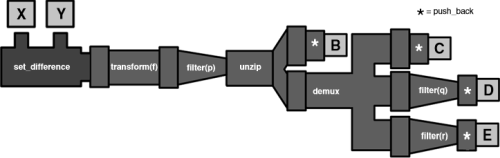Figure 2
```  std::set_difference(begin(setA), end(setA),
begin(setB), end(setB),
transform(f) >>= filter(p)

## Streams support

The contents of an input stream can be sent to a pipe by using ``` read_in_stream ``` . The end pipe ``` to_out_stream ``` sends data to an output stream.

The following example reads strings from the standard input, transforms them to upper case, and sends them to the standard output:

```  std::cin >>= pipes::read_in_stream<std::string>{}
>>= pipes::transform(toUpper)
>>= pipes::to_out_stream(std::cout);```

## General pipes

### demux``` demux ``` is a pipe that takes any number of pipes, and sends a copy of the values it receives to each of those pipes.

```  std::vector<int> input = {1, 2, 3,
4, 5};
std::vector<int> results1;
std::vector<int> results2;
std::vector<int> results3;

input >>= pipes::demux(
pipes::push_back {results1),
pipes::push_back(results2),
pipes::push_back(results3));
// results1 contains {1, 2, 3, 4, 5}
// results2 contains {1, 2, 3, 4, 5}
// results3 contains {1, 2, 3, 4, 5}```

### dev_null

``` dev_null ``` is a pipe that doesn’t do anything with the value it receives. It is useful for selecting only some data coming out of an algorithm that has several outputs. An example of such algorithm is ``` set_segregate ``` [ Boccara ]:

```  std::set<int> setA = {1, 2, 3, 4, 5};
std::set<int> setB = {3, 4, 5, 6, 7};
std::vector<int> inAOnly;
std::vector<int> inBoth;
sets::set_seggregate(setA, setB,
pipes::push_back(inAOnly),
pipes::push_back(inBoth),
dev_null{});
// inAOnly contains {1, 2}
// inBoth contains {3, 4, 5}```

### drop

``` drop ``` is a pipe that ignores the first N incoming values, and sends on the values after them to the next pipe:

```  auto const input = std::vector<int>{ 1, 2, 3, 4,
5, 6, 7, 8, 9, 10};
auto result = std::vector<int>{};
input >>= pipes::drop(5)
>>= pipes::push_back(result);
// result contains { 6, 7, 8, 9, 10 }```

### drop_while

``` drop ``` is a pipe that ignores the incoming values until they stop satisfying a predicate, and sends on the values after them to the next pipe:

```  auto const input = std::vector<int>{ 1, 2, 3, 4,
5, 6, 7, 8, 9, 10};
auto result = std::vector<int>{};
input >>= pipes::drop_while([](int i)
{ return i != 6; })
>>= pipes::push_back(result);
// result contains { 6, 7, 8, 9, 10 }```

### filter``` filter ``` is a pipe that takes a predicate ``` p ``` and, when it receives a value ``` x ``` , sends the result on to the next pipe if ``` p(x) ``` is ``` true ``` .

```  std::vector<int> input = {1, 2, 3, 4, 5, 6, 7, 8,
9, 10};
std::vector<int> results;
input >>= pipes::filter([](int i)
{ return i % 2 == 0; })
>>= pipes::push_back(results);
// results contains {2, 4, 6, 8, 10}```

### join

The ``` join ``` pipe receives collection and sends each element of each of those collections to the next pipe:

```  auto const input = std::vector<std::vector<int>>{
{1, 2}, {3, 4}, {5, 6} };
auto results = std::vector<int>{};
input >>= pipes::join
>>= pipes::push_back(results);
// results contain {1, 2, 3, 4, 5, 6}```

### partition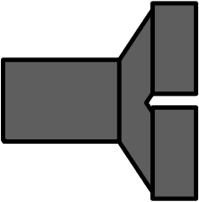``` partition ``` is a pipe that takes a predicate ``` p ``` and two other pipes. When it receives a value ``` x ``` , sends the result on to the first pipe if ``` p(x) ``` is ``` true ``` , and to the second pipe if ``` p(x) ``` is ``` false ``` .

```  std::vector<int> input = {1, 2, 3,
4, 5, 6, 7, 8, 9, 10};
std::vector<int> evens;
std::vector<int> odds;
input >>= pipes::partition([](int n)
{ return n % 2 == 0; },
pipes::push_back(evens),
pipes::push_back(odds));
// evens contains {2, 4, 6, 8, 10}
// odds contains {1, 3, 5, 7, 9}```

``` read_in_stream ``` is a template pipe that reads from an input stream. The template parameter indicates what type of data to request from the stream:

```  auto const input = std::string{"1.1 2.2 3.3"};
std::istringstream(input)
>>= pipes::transform([](double d)
{ return d * 10; })
>>= pipes::push_back(results);
// results contain {11, 22, 33};```

### switch

``` switch_ ``` is a pipe that takes several ``` case_ ``` branches. Each branch contains a predicate and a pipe. When it receives a value, it tries it successively on the predicates of each branch, and sends the value on to the pipe of the first branch where the predicate returns ``` true ``` . The ``` default_ ``` branch is equivalent to one that takes a predicate that returns always ``` true ``` . Having a ``` default_ ``` branch is not mandatory.

```  std::vector<int> numbers = {1, 2, 3, 4, 5, 6, 7,
8, 9, 10};
std::vector<int> multiplesOf4;
std::vector<int> multiplesOf3;
std::vector<int> rest;
numbers >>= pipes::switch_(
pipes::case_([](int n){ return n % 4 == 0; })
>>= pipes::push_back(multiplesOf4),
pipes::case_([](int n){ return n % 3 == 0; })
>>= pipes::push_back(multiplesOf3),
pipes::default_ >>= pipes::push_back(rest) ));
// multiplesOf4 contains {4, 8};
// multiplesOf3 contains {3, 6, 9};
// rest contains {1, 2, 5, 7, 10};```

### take

``` take ``` takes a number ``` N ``` and sends to the next pipe the first ``` N ``` element that it receives. The elements after it are ignored:

```  auto const input = std::vector<int>{1, 2, 3, 4,
5, 6, 7, 8, 9, 10};
auto result = std::vector<int>{};
input >>= pipes::take(6)
>>= pipes::push_back(result);
// result contains {1, 2, 3, 4, 5, 6}```

### take_while

``` take_while ``` takes a predicate and sends to the next pipe the first values it receives. It stops when one of them doesn’t satisfy the predicate:

```  auto const input = std::vector<int>{1, 2, 3, 4,
5, 6, 7, 8, 9, 10};
auto result = std::vector<int>{};
input >>= pipes::take_while([](int i){
return i != 7; })
>>= pipes::push_back(result);
// result contains {1, 2, 3, 4, 5, 6}```

### tee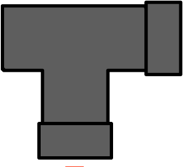``` tee ``` is a pipe that takes one other pipe, and sends a copy of the values it receives to each of these pipes before sending them on to the next pipe. Like the ``` tee ``` command on UNIX, this pipe is useful to take a peek at intermediary results.

```  auto const inputs = std::vector<int>{1, 2, 3, 4,
5, 6, 7, 8, 9, 10};
auto intermediaryResults = std::vector<int>{};
auto results = std::vector<int>{};
inputs >>= pipes::tee(pipes::push_back
(intermediaryResults))
>>= pipes::push_back(results);
// intermediaryResults contains {2, 4, 6, 8, 10,
// 12, 14, 16, 18, 20}
// results contains {12, 14, 16, 18, 20}```

### transform``` transform ``` is a pipe that takes a function ``` f ``` and, when it receives a value, applies ``` f ``` on it and sends the result on to the next pipe.

```  std::vector<int> input = {1, 2, 3, 4, 5};
std::vector<int> results;
input >>= pipes::transform([](int i)
{ return i*2; })
>>= pipes::push_back(results);
// results contains {2, 4, 6, 8, 10}```

### unzip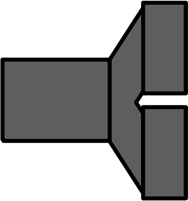``` unzip ``` is a pipe that takes N other pipes. When it receives a ``` std::pair ``` or ``` std::tuple ``` of size N (for ``` std::pair ``` N is 2), it sends each of its components to the corresponding output pipe:

```  std::map<int, std::string> entries = { {1,
"one"}, {2, "two"}, {3, "three"}, {4, "four"},
{5, "five"} };
std::vector<int> keys;
std::vector<std::string> values;
entries >>= pipes::unzip(pipes::push_back(keys),
pipes::push_back(values)));
// keys contains {1, 2, 3, 4, 5};
// values contains {"one", "two", "three", "four",
// "five"};```

## End pipes

### custom

``` custom ``` takes a function (or function object) that sends to the data it receives to that function. One of its usages is to give legacy code that does not use STL containers access to STL algorithms:

```  std::vector<int> input = {1, 2, 3, 4, 5, 6, 7 ,8,
9, 10};
void legacyInsert(int number, DarkLegacyStructure
const& thing); // this function inserts into
// the old non-STL container
DarkLegacyStructure legacyStructure = // ...

std::copy(begin(input), end(input),
custom([&legacyStructure](int number){
legacyInsert(number, legacyStructure); });```

Read the full story about making legacy code compatible with the STL on my blog [ Boccara17a ].

Note that ``` custom ``` goes along with a helper function object, ``` do_ ``` , that allows to perfom several actions sequentially on the output of the algorithm:

```  std::copy(begin(input), end(input),
pipes::custom(pipes::do_([&](int i){
results1.push_back(i*2);}).
then_([&](int i){ results2.push_back(i+1);}).
then_([&](int i){ results3.push_back(-i);})));```

### map_aggregator

``` map_aggregator ``` provides the possibility to embark an aggregator function in the inserter iterator, so that new elements whose key is already present in the map can be merged with the existent (e.g. have their values added together).

```  std::vector<std::pair<int, std::string>> entries
= { {1, "a"}, {2, "b"}, {3, "c"}, {4, "d"} };
std::vector<std::pair<int, std::string>> entries2
= { {2, "b"}, {3, "c"}, {4, "d"}, {5, "e"} };
std::map<int, std::string> results;
std::copy(entries.begin(), entries.end(),
map_aggregator(results, concatenateStrings));
std::copy(entries2.begin(), entries2.end(),
map_aggregator(results, concatenateStrings));
// results contains { {1, "a"}, {2, "bb"},
// {3, "cc"}, {4, "dd"}, {5, "e"} }```

``` set_aggreagator ``` provides a similar functionality for aggregating elements into sets.

Read the full story about ``` map_aggregator ``` and ``` set_aggregator ``` on my blog [ Boccara17b ].

### override

``` override ``` is the pipe equivalent to calling ``` begin ``` on an existing collection. The data that ``` override ``` receives overrides the first element of the container, then the next, and so on:

```  std::vector<int> input = {1, 2, 3, 4, 5, 6, 7, 8,
9, 10};
std::vector<int> results = {0, 0, 0, 0, 0, 0, 0,
0, 0, 0};
input >>= pipes::filter([](int i)
{ return i % 2 == 0; })
>>= pipes::override(results);
// results contains {2, 4, 6, 8, 10, 0, 0, 0, 0, 0};```

### push_back

``` push_back ``` is a pipe that is equivalent to ``` std::back_inserter ``` . It takes a collection that has a ``` push_back ``` member function, such as a ``` std::vector ``` , and ``` push_back ``` s the values it receives into that collection.

### set_aggregator

Like ``` map_aggregator ``` , but inserting/aggregating into ``` std::sets ``` . Since ``` std::set ``` values are const, this pipe erases the element and re-inserts the aggregated value into the ``` std::set. ```

```  struct Value
{
int i;
std::string s;
};
bool operator==(Value const& value1,
Value const& value2)
{
return value1.i == value2.i && value1.s
== value2.s;
}
bool operator<(Value const& value1,
Value const& value2)
{
if (value1.i < value2.i) return true;
if (value2.i < value1.i) return false;
return value1.s < value2.s;
}
Value concatenateValues(Value const& value1,
Value const& value2)
{
if (value1.i != value2.i) throw
std::runtime_error("Incompatible values");
return { value1.i, value1.s + value2.s };
}
int main()
{
std::vector<Value> entries = { Value{1, "a"},
Value{2, "b"}, Value{3, "c"}, Value{4, "d"} };
std::vector<Value> entries2 = { Value{2, "b"},
Value{3, "c"}, Value{4, "d"}, Value{5, "e"} };
std::set<Value> results;
std::copy(entries.begin(), entries.end(),
pipes::set_aggregator(results,
concatenateValues));
std::copy(entries2.begin(), entries2.end(),
pipes::set_aggregator(results,
concatenateValues));
// results contain { Value{1, "a"}, Value{2,
// "bb"}, Value{3, "cc"}, Value{4, "dd"},
// Value{5, "e"} }
}```

### sorted_inserter

In the majority of cases where it is used in algorithms, ``` std::inserter ``` forces its user to provide a position. It makes sense for un-sorted containers such as ``` std::vector ``` , but for sorted containers such as ``` std::set ``` , we end up choosing begin or end by default, which doesn’t make sense:

```  std::vector<int> v = {1, 3, -4, 2, 7, 10, 8};
std::set<int> results;
std::copy(begin(v), end(v),
std::inserter(results, end(results)));```

``` sorted_inserter ``` removes this constraint by making the position optional. If no hint is passed, the container is left to determine the correct position to insert:

```  std::vector<int> v = {1, 3, -4, 2, 7, 10, 8};
std::set<int> results;
std::copy(begin(v), end(v),
sorted_inserter(results));
//results contains { -4, 1, 2, 3, 7, 8, 10 }```

Read the full story about ``` sorted_inserter ``` on my blog [ Boccara17c ].

### to_out_stream

``` to_out_stream ``` takes an output stream and sends incoming to it:

```  auto const input =
std::vector<std::string>{"word1", "word2",
"word3"};
input >>= pipes::transform(toUpper)
>>= pipes::to_out_stream(std::cout);
// sends "WORD1WORD2WORD3" to the standard output```

## References

[Boccara] Jonathan Boccara, ‘Sets’, on https://github.com/joboccara/sets

[Boccara17a] Jonathan Boccara, ‘How to Use the STL With Legacy Output Collections’, published 24 November 2017, available at https://www.fluentcpp.com/2017/11/24/how-to-use-the-stl-in-legacy-code/

[Boccara17b] Jonathan Boccara, ‘A smart iterator for aggregating new elements with existing ones in a map or a set’, published 21 March 2017, available at https://www.fluentcpp.com/2017/03/21/smart-iterator-aggregating-new-elements-existing-ones-map-set/

[Boccara17c] Jonathan Boccara, ‘A smart iterator for inserting into a sorted container in C++’, published 17 March 2017, available at https://www.fluentcpp.com/2017/03/17/smart-iterators-for-inserting-into-sorted-container/